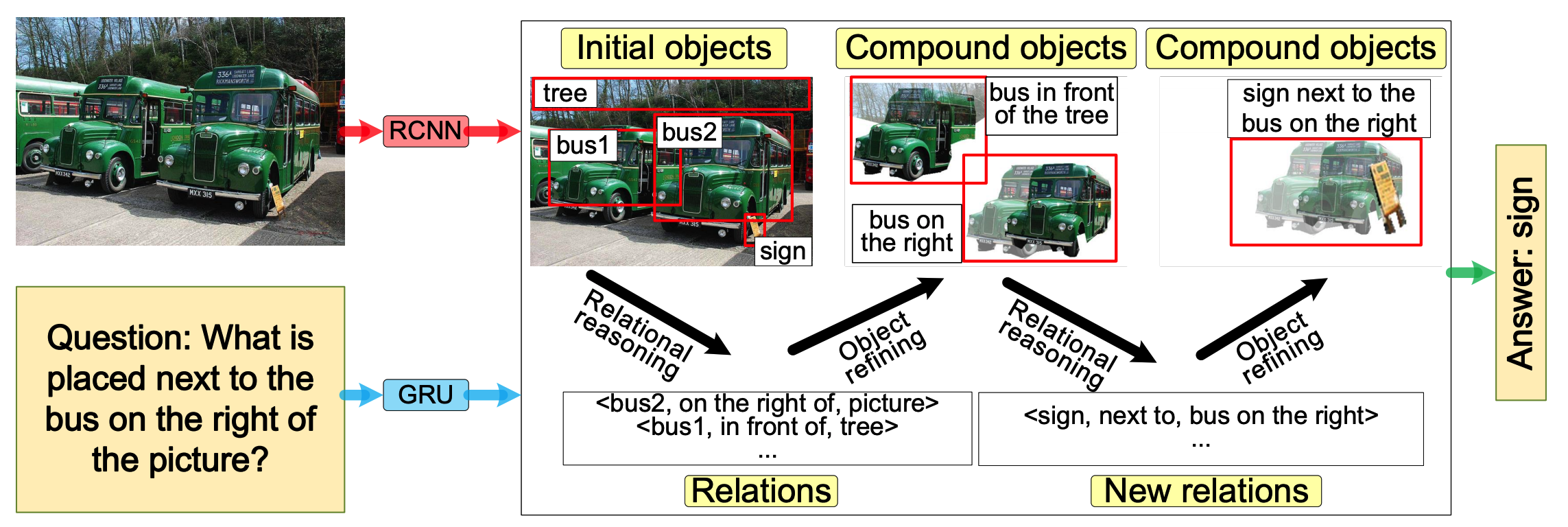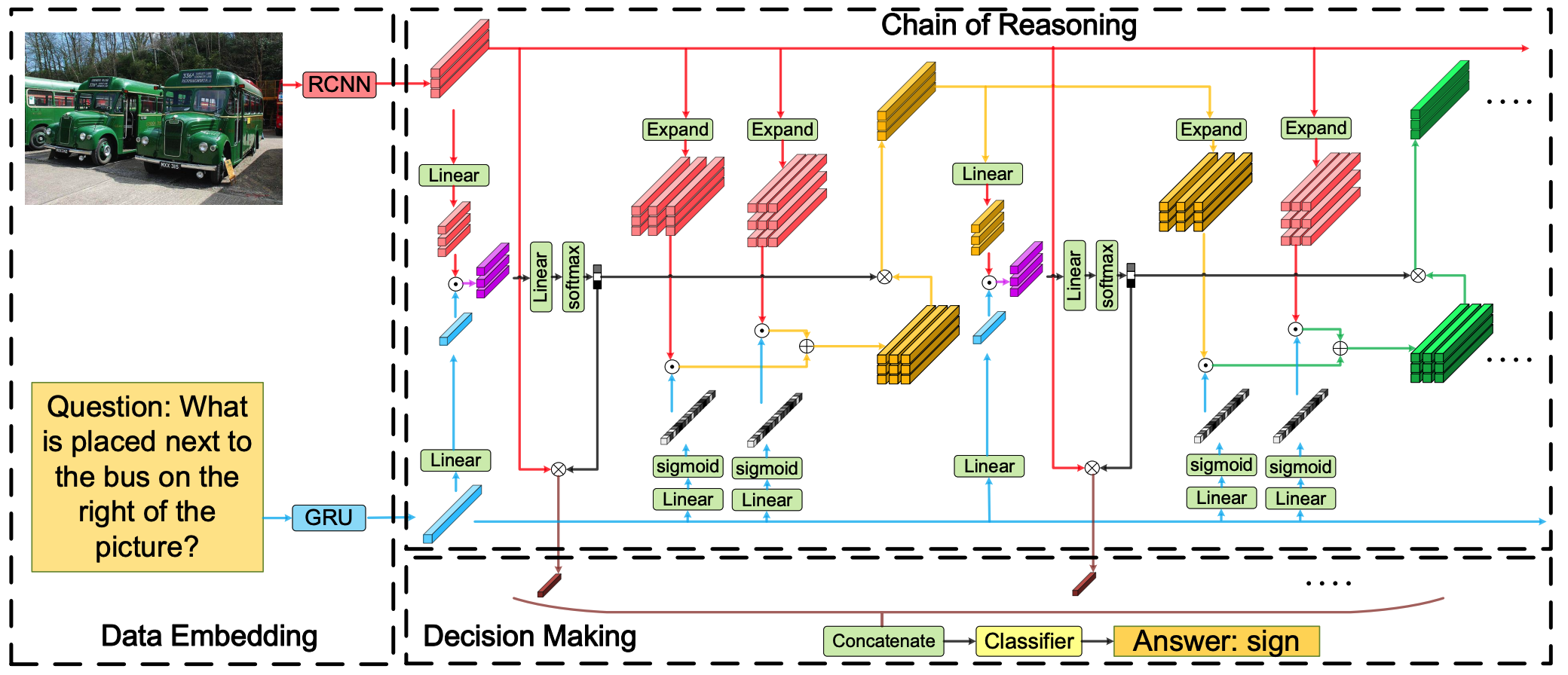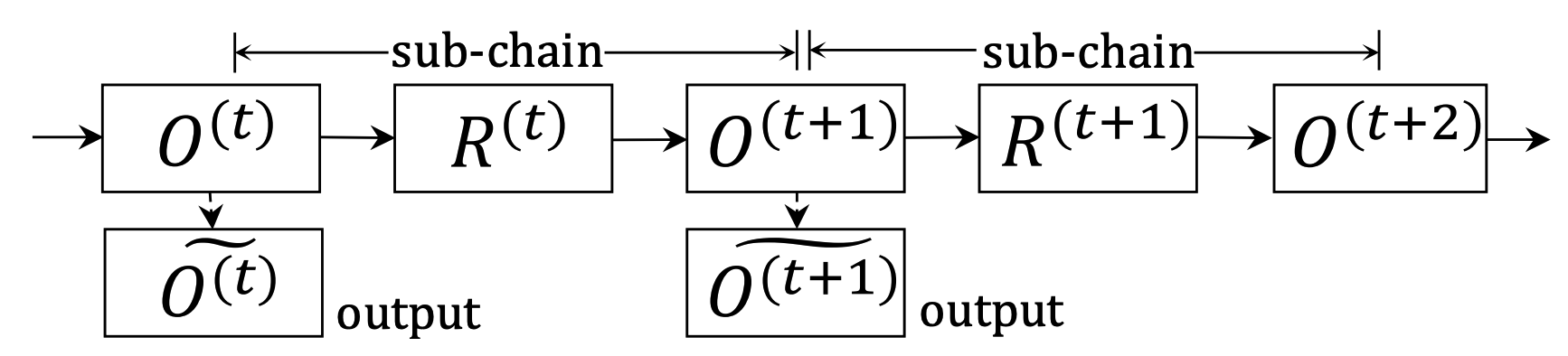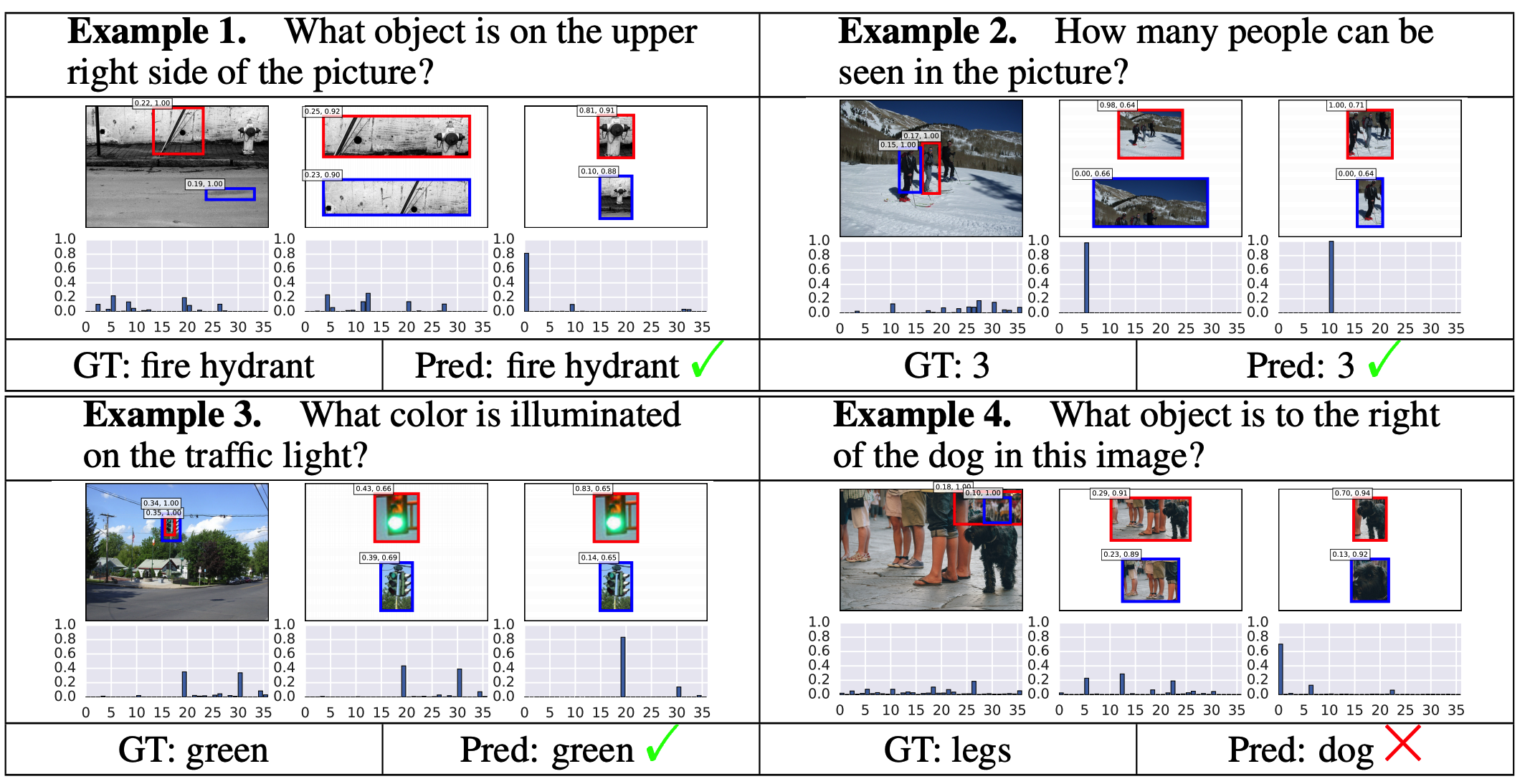# Chain of Reasoning for Visual Question Answering

## WHY?

Previous methods for visual question answering performed one-step or static reasoning while some questions requires chain of reasonings.

## WHAT?Chain of reasoning(CoR) model alternatively updates the objects and their relations to solve questions that require chain of reasoning.Cor consists of three parts: Data embedding, chain of reasoning and decision making. Data embedding encodes images and questions into vecors with Faster-RCNN and GRU respectively.Chain of reasoning step consists of a series of sub-chains that perform relational reasoning and object refining. Given that objects of image denoted by O^{(t)}$O^{(t)}$, output is calculated as follows.

P^{t} = relu(o^{t}W_o^{t}), S^{t} = relu(QW_q^{t})\\
F^{t} = \sum_{k=1}^K(P^{t}W_{p, k}^{t})\odot(S^{t}W_{s, k})\\
\alpha^{t} = softmax(F^{t}W_f^{t})\\
\tilde{Q}^{t} = (\alpha^{t})^T O^{t}

Relations of objects are calculated with guidance conditioned on question. Objects are refined with weighted sum of relations.

G_l = \sigma(relu(QW_{l_1})W_{l_2}), G_r = \sigma(relu)QW_{r_1})W_{r_2}\\
R_{ij}^{t} = (O_i^{t}\odot G_l) \oplus (O_j^{(1)}\odot G_r)\\
O_j^{t+1} = \sum_{i=1}^m \alpha_i^t R_{ij}^t

Decisions are made with all the objects from each step.

O^* = [relu(O^{1}W^{1});relu(O^{2}W^{2});...;relu(O^{T}W^{T})\\
H = \sum_{k=1}^K(O^* W_{O^*, k})\odot(QW_{q', k})\\
\hat{a} = softmax(HW_h)

## So?CoR achieved the best result on various VQA tasks including VQA 1.0, VQA 2.0, COCO-QA, and TDIUC. Visualization shows CoR performs appropriate reasoning.

## Critic

I think more explanation is needed in architecture of relational reasoning and object refining step. Also, performance difference in ablation studies seems too trivial to draw conclusion.

Powered by aiden